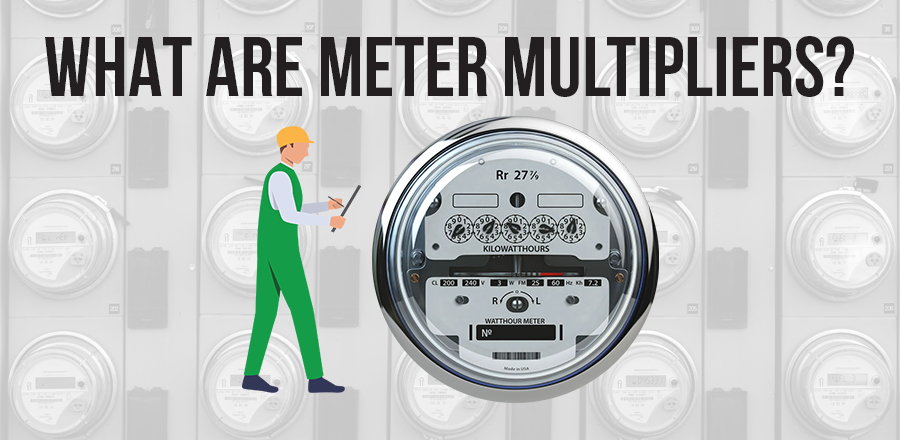June 27, 2022###### MRI Ascend 2022 Event
September 27, 2022June 27, 2022###### MRI Ascend 2022 Event
September 27, 2022The meter multiplier times the change in beginning and ending meter readings is equal to the actual energy use

# Meter Multipliers & Other Meter Issues

Meter Multipliers – Every meter has one. Some meters have two different ones.  This discussion is to help you understand a meter multiplier.

What are they? A meter multiplier is the basic unit of measurement for a particular meter.  In decimal math, that means the digit to the furthest right that actually moves.  Here are some examples:

• 000432 OO – the last moving digit is the 2. What decimal place does the last moving digit fall into?
• 005539 O – the last moving digit is the 9. What decimal place does the last moving digit fall into?
• 0067841.12 – the last moving digit is the 2. What decimal place does the last moving digit fall into?

What does billing do? Billing will seek to convert a reading (or set of readings) to a unit of measurement that is suited for rate calculations, and one that the general public is familiar with.  As an example, a meter reading representing 100 gallons will be converted to single gallons, which allows the public to more accurately understand their usage.  Using the readings above, this would be as follows:

• 00043200
• 0055390
• 0067841

Electronic versus meter face – there are some occasions where the meter face reads in one unit of measurement and the electronic output in another. A common example is a water meter that reads in tenth or hundredth gallon on the face. The electronic output of a water meter is usually in ten-gallon increments.  The following would be true:

• Face - 0067841.12
• Transmitter - 6784

Notice that 1.12 does not register on the transmitter.  The transmitter is reading in ten-gallon increments, so anything beyond the tens digit is pure speculation.

Billing calculations – the billing department calculates usage in gallons by taking the ending reading, minus the start reading, and multiplying the result by 10. That would look like this: